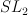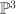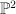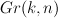Posts tagged ‘Imperial’

## V. Batyrev: “Toric Deformations of Some Spherical Fano Varieties”

Here are my (incomplete) notes from Batyrev’s talk in the Extremal Laurent Polynomials workshop at Imperial:

Batyrev_London_Sep_2010

If you have complete notes, please post them here.

Partial summary:

• spherical varieties
• a model family of examples:$SL_2$-varieties
• review: compactification and toric varieties
• spherical compactification in these examples
• valuations and coloured cones
• finding toric degenerations

## K. Altmann: “Deformations of Gorenstein Canonical Toric Singularities”

Here are my notes from Altmann’s talk at the Extremal Laurent Polynomials workshop at Imperial:

Altmann_London_Sep_2010

Summary:

• an example due to Pinkham
• deformations and Minkoski sums; a lattice condition
• constructing the deformation corresponding to a Minkwoski decomposition
• the versal deformation space and the moduli space of generalized Minkowski summands of Q
• Example: the cone over a hexagon
• the equations defining the versal deformation space in the isolated Gorenstein case
• a new point of view: double divisors

## B. Siebert: “A Tropical View on Landau-Ginzburg Models”

Here are my notes from Siebert’s talk at the Extremal Laurent Polynomials workshop at Imperial:

Siebert_London_Sep_2010

Summary:

• An overview of toric degenerations and the Gross–Siebert picture
• Examples: a pencil of quartics in$\PP^3$ and a pencil of elliptic curves in$\PP^2$
• the Gross–Siebert Reconstruction Theorem
• Mirror Symmetry and the discrete Legendre Transform
• Landau–Ginzburg models; the Hori–Vafa mirror
• how to extend the superpotential from the central fiber to the whole of the mirror family in such a way that the resulting superpotential is proper
• Example:$\PP^2$, flattening the boundary of the polyhedral complex
• Broken lines and scattering

## V. Golyshev: “The Apery Class and the Gamma Class”

Here are my notes from Golshev’s talk at the Extremal Laurent Polynomials workshop at Imperial:

Golyshev_London_Sep_2010

Summary:

• a historical analogy: the study of Fano varieties now versus the study of algebraic varieties in the early 1970s
• the key idea: classifying Fanos by detecting and classifying Fano quantum motives and their realizations
• possible approaches
• the Tannakian picture
• the quantum Satake correspondence (Golyshev–Manivel)
• two steps in this direction:  Ueda’s proof of the Dubrovin Conjecture for$Gr(k,n)$; Galkin–Golyshev–Iritani’s proof that Apery=Gamma
• irregular monodromy data for Dubrovin’s quantum connection
• the Extended Dubrovin Conjecture and the Apery=Gamma hypothesis
• the Extended Dubrovin Conjecture and the Apery=Gamma hypothesis hold for$Gr(k,n)$